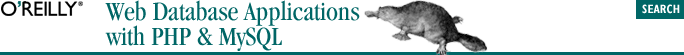home | O'Reilly's CD bookshelfs | FreeBSD | Linux | Cisco | Cisco Exam## 2.5. Arrays

In PHP, an array can hold scalar values—integers, Booleans, strings, or floats—or compound values—objects and even other arrays, and can hold values of different types. In this section, we show how arrays are constructed and introduce several useful array functions from the PHP library.

### 2.5.5.1. Sorting with sort( ) and rsort( )

```sort(array subject [, integer sort_flag])
rsort(array subject [, integer sort_flag])```

Both functions sort the subject array based on the values of each element. The following example shows the sort( ) function on an array of integers:

```\$numbers = array(24, 19, 3, 16, 56, 8, 171);
sort(\$numbers);

foreach(\$numbers as \$n)
echo \$n . " ";```

The output of the example prints the elements sorted by value:

`3 8 16 19 24 56 171`

Another way to examine the contents of the sorted array is to use the print_r( ) function described in Section 2.1.7. The output of the statement print_r(\$numbers) shows the sorted values with the associated index:

```Array (  => 3
 => 8
 => 16
 => 19
 => 24
 => 56
 => 171 ) ```

The following example shows the rsort( ) function on the same array:

```\$numbers = array(24, 19, 3, 16, 56, 8, 171);
rsort(\$numbers);
print_r(\$numbers);```

The output of the example shows the elements sorted in reverse order by value:

```Array (  => 171
 => 56
 => 24
 => 19
 => 16
 => 8
 => 3 ) ```

By default, PHP sorts strings in alphabetical order and numeric values in numeric order. An optional parameter, sort_flag, can be passed to force the string or numeric sorting behavior. In the following example, the PHP constant SORT_STRING sorts the numbers as if they were strings:

```\$numbers = array(24, 19, 3, 16, 56, 8, 171);
sort(\$numbers, SORT_STRING);
print_r(\$numbers);```

The output of the example shows the result:

```Array (  => 16
 => 171
 => 19
 => 24
 => 3
 => 56
 => 8 ) ```

Many of the array sorting functions accept a sort_flag parameter. Other sort flags are SORT_REGULAR to compare items in the array normally and SORT_NUMERIC that forces items to be compared numerically.

sort( ) and rsort( ) can be used on associative arrays, but the keys are lost. The resulting array contains only the values in the sorted order. Consider the following example:

```\$map =
array("o"=>"kk", "e"=>"zz", "z"=>"hh", "a"=>"rr");

sort(\$map);
print_r(\$map);```

The print_r( ) output shows the modified array without the key values:

`Array (  => hh  => kk  => rr  => zz ) `

### 2.5.5.4. Sorting with user-defined element comparison

```usort(array subject, string compare_function)
uasort(array subject, string compare_function)
uksort(array subject, string compare_function)```
`integer my_compare_function(mixed a, mixed b)`

We discuss how to write functions in more detail in Section 2.10. The compare function takes two arguments, a and b, and returns -1 if a is less than b, 1 if a is greater than b, and 0 if a and b are equal. How the function determines that one value is less than, greater than, or equal to another depends on the requirements of sorting. The following example shows how usort( ) sorts an array of strings based on the length of each string:

```// Compare two string values based on the length
function cmp_length(\$a, \$b)
{
if (strlen(\$a) < strlen(\$b)) return -1;
if (strlen(\$a) > strlen(\$b)) return 1;

// String lengths must be equal
return 0;
}

\$animals =
array("cow", "ox", " hippopotamus", "platypus");

usort(\$animals, "cmp_length");

print_r(\$animals);```

The array \$animals is printed:

`Array (=>ox =>cow =>platypus =>hippopotamus)`